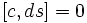# Invariance under any set of derivations is centralizer-closed

## Statement

### For a single derivation

Suppose$L$ is a Lie ring,$S$ is a subring of a Lie ring, and$d$ is a derivation of$L$ such that$d(S) \subseteq S$. Let$C = C_L(S)$ be the centralizer of$S$. Then,$C$ is also invariant under$d$.

### For a bunch of derivations

Suppose$L$ is a Lie ring,$S$ is a subring of a Lie ring, and$D$ is a set of derivations of$L$ such that$d(S) \subseteq S$ for all$d \in D$. Let$C = C_L(S)$ be the centralizer of$S$. Then,$C$ is also invariant under all$d \in D$.

## Proof

Note that the statements for a single derivatoin and for a bunch of derivations are clearly equivalent, so we only prove the former.

Given: A Lie ring$L$, a subring$S$ of$L$.$C = C_L(S)$ is the set of$a \in L$ such that$[a,s] = 0$ for all$s \in S$. A derivation$d$ of$L$ such that$d(S) \subseteq S$.

To prove:$d(C) \subseteq C$.

Proof: For any$c \in C$ and$s \in S$, we need to show that$[dc,s] = 0$.

For this, note that, by the Leibniz rule property of derivations:$d([c,s]) = [dc,s] + [c,ds]$.

Since$[c,s] = 0$, the left side is zero. Further, since$d(S) \subseteq S$,$ds \in S$, so$[c,ds] = 0$. This gives$[dc,s] = 0$ as required.